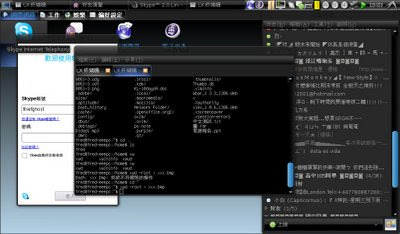## 發表文章

### 一年一度的 COSCUP 又夠來囉

。而你當初之所以選用Ａ軟體而不是Ｂ軟體，常常是聽信銷售員（sales）的一面之詞或同儕

，users 遇到的問題要間接地才能反應到 coders 手上，中間還可能會被過濾掉。

### 用 C 語言實作矩陣運算的 Function

typedef struct {
int rows;
int cols;
int total;
double *table;
} Matrix;

Matrix *matrix_create(int rows, int cols)

Matrix *matrix_optimize(Matrix *matrix)

Matrix *matrix_solution(Matrix *matrix)

void matrix_print(Matrix *matrix)

void matrix_clear(Matrix *matrix)

void matrix_clear(Matrix *matrix)
{
int i, j;
double *ptr;

/* initializing matrix */
ptr = matrix->table;
for (i=0;i<matrix->total;i++)
*(ptr++) = 0;
}

Matrix *matrix_create(int rows, int cols)
{
Matrix *matrix;

/* allocate */
matrix = (Matrix *)malloc(sizeof(Matrix));
matrix->rows = rows;
matrix->cols = cols;
matrix->total = rows * cols…

### 判別WEP的ASCII與Hex型態之密碼

40bits 用 ASCII 表示：
iwconfig wifi0 key "s:12345"

104bits 用 ASCII 表示：
iwconfig wifi0 key "s:1234567890abc"

40bits 用 16 進位表示：
iwconfig wifi0 key "AABBCCDDEE"

104bits 用 16 進位表示：
iwconfig wifi0 key "AABBCCDDEEFF11223344556677"

### 開發時面對 GTK+ 臭蟲的一點小技巧

GTK+』是個易學的 Toolkit，用它來寫 Linux/BSD 上的圖形化界面，非常的簡單。用其開發出來的程式，除了可以在 X11 上運作，甚至是可以使用 DirectFB 直接將 GTK+ 程式畫在 FrameBuffer 上，所以 GTK+ 也常見於 Embedded System。

G_DEBUG="fatal-criticals" 在產生 Makefiles 時，可下參數開啟 cast-checks 以得到更多的 debug information：
./configure --enable-cast-checks 用這幾個小技巧，就能比較容易發現 GTK+ 程式的錯誤。

Open Source 最大的好處是讓開發者可以到處看 Code ，這意味著一切技術都是透明的，對於提升開發者自身能力上是很棒的。而對使用很多不同 Libraries 的 Project 來說，如果在有許多樣本的情況下，其開發者看 Code 的數量，其實就是程式碼品質的關鍵。所以，GTK+ 的 Source Code 當然也要看一下才是。 :D

### 成為俠侶 LXPC．讓 LXDE 與低價電腦雙宿雙飛EeePC 900 實體跑 LXDE

LXDE 下一階段目標是實作許多『好用』的系統工具和設定工具，如螢幕/投影機設定工具、網路連線工具、偏好設定等等；此外，也許會花些時間實作和研究一些 eye-candy 的東西，不過暫時不會當成主要工作，主要目標還是放在提升 LXDE 的可用性。

LXTerminal 目前特色：
* 支援多重分頁。
* 沒有任何過度不必要的依賴套件。〔除了 gtk+ 相關之外，就只有 libvte〕
* 為減少記憶體浪費，所有的程式運作可共用一個 process。
* 當視窗、分頁和 VTE 的尺寸改變時，有正確的變化行為且處理效能良好。
* 支援共用 process時，使用速度極快的原生 Unix-socket 而不使用 D-bus。

### GLib 就是懶．為一些 G stuffs 加上多國語言支援

{
{ N_("/_File"), NULL, NULL, 0, "<branch>" },
{ N_("/_File/_New Window"), NEW_WINDOW_ACCEL, newwindow, 1, "<stockitem>", GTK_STOCK_ADD }
};

N_…

### 新專案 LXTerminal 和 LXLauncher2 嘗鮮！EeePC 上的 LXDE

### 頭痛．驚見．回憶予我### GLib 就是懶．用 GKeyFile 存取設定檔

Glib Utilities 中有個函式包裝『GKeyFile』，此包裝提供一個存取設定檔的界面，讓程式開發者不再需要為了讀取設定檔，而被迫自行撰寫許多程式來處理檔案資料流和分析設定檔，是個非常省時省力的函式包裝。

GKeyFile 存取的設定檔，就是一個 .ini 檔的形式，大致上像：
[group1]
mykey1=66
mykey2=Hello World

[group2]
yourkey1=yes
yourkey2=3.1415926

#include <stdio.h>
#include <glib.h>

void main(void)
{
GKeyFile *keyfile;
GKeyFileFlags flags;
GError *error = NULL;

/* 初始化 GKeyFile */
keyfile = g_key_file_new();

/* 讀取 config file */
if (!g_key_file_load_from_file (keyfile, "helloworld.conf", flags, &error))
return;

/* 讀取設定並顯示 */
printf("[Group1]\n");
printf("mykey1: %d\n", g_key_file_get_integer(keyfile, "group1", "mykey1", NULL));
p…

### 使用 C 語言做『梯型法則』積分運算

『梯型法則 Trapezoidal Rule』是積分方法的基本定理之一，從高中到大學的數學課程中都有提到，碰到工程這種需要大量求值的領域，我們就必需將這些積分方法寫成程式。

#include <stdio.h>
#include <math.h>

double my_eq_orig(double x)
{
return 0.2 + 25*x - 200*pow(x, 2) + 675*pow(x, 3) - 900*pow(x, 4) + 400*pow(x, 5);
}

double my_eq(double x)
{
return -400 + 4050*x - 10800*pow(x, 2) + 8000*pow(x,3);
}

double integrate_trapezoidal(double (*fx)(double), int n, double a, double b)
{
int i;
double h;
double x = a;
double s = 0;

h = (b - a) / n;
for (i=1;i<=n-1;i++) {
x += h;
s = s + fx(x);
}

return h * ((fx(a) + fx(b)) / 2 + s);
}

void main(void)
{
/* real */
double real = integrate_trapezoidal(my_eq_orig, 100, 0, 0.8);
/* n = 1 */
double real_i = integrate_trapezoidal(my_eq_orig, 1, 0, 0.8);
/* average */
double fa = integrate_trapezoidal(my_eq, 100, 0, 0.8) / 0.8;

printf("real value:\n%lf\n\n", real);
printf("I:\n%lf\n\n", real_i);
printf("f(x)\'\' average:\…

### 一朝被蛇咬，十年怕草繩